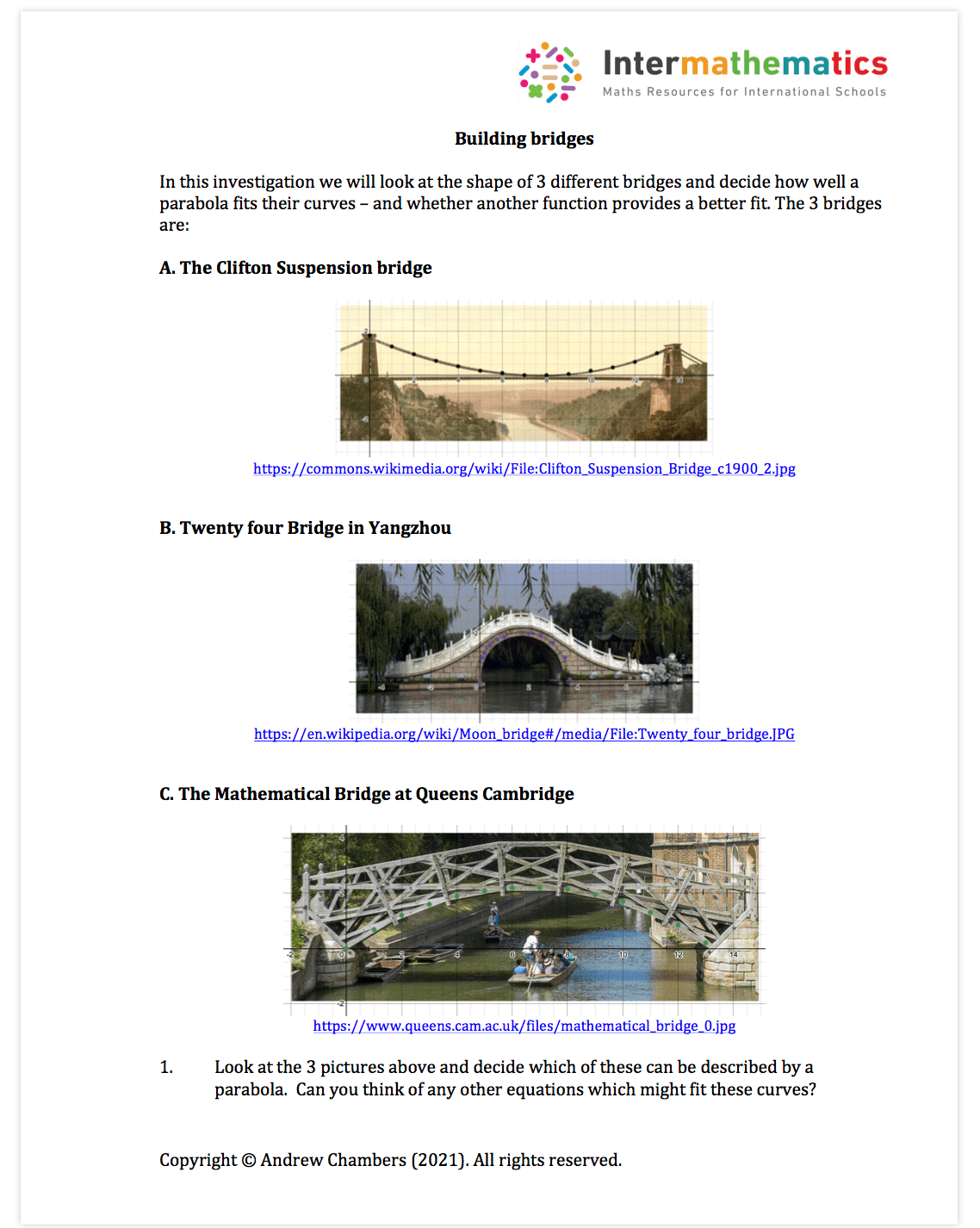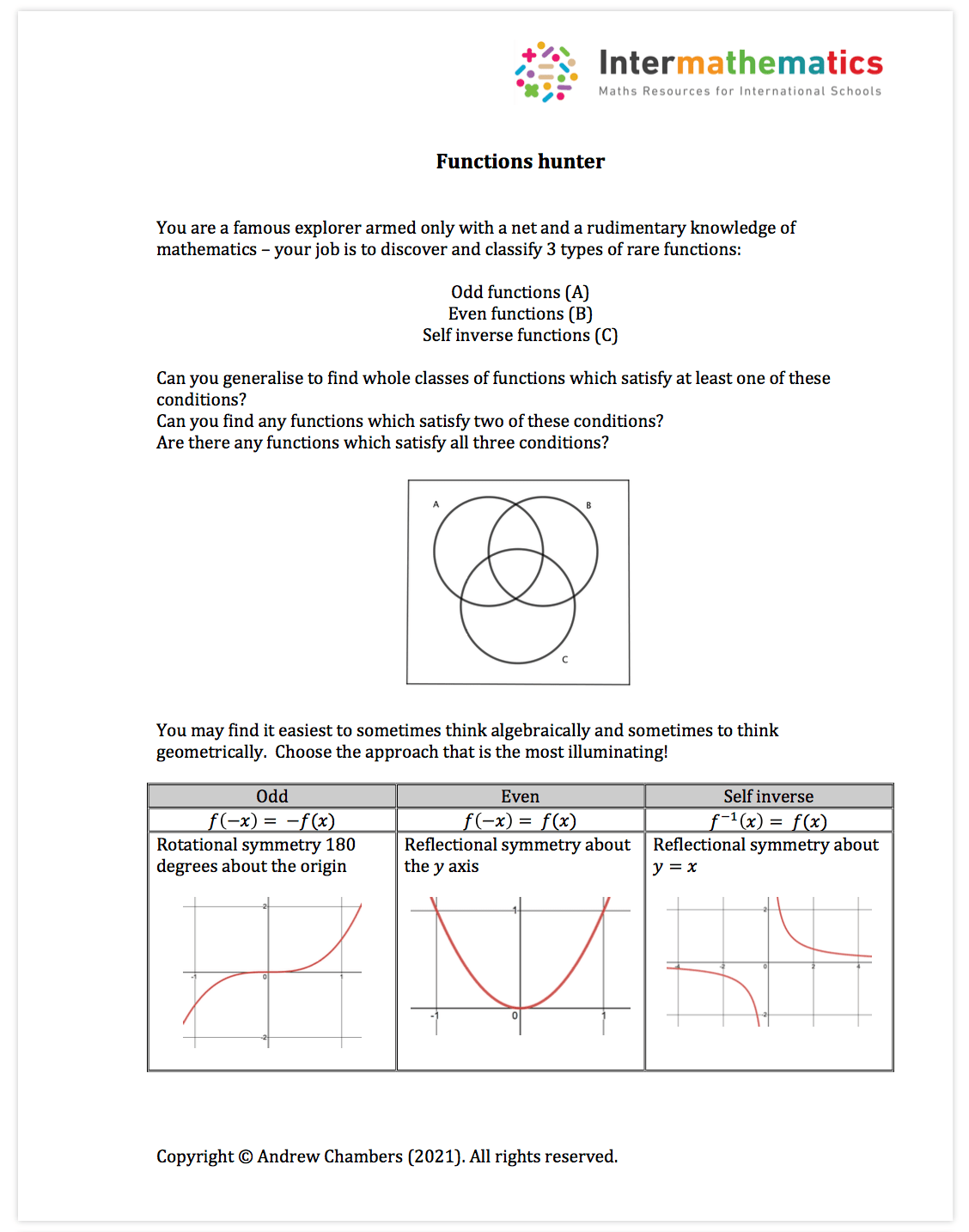# Functions Free Content

Functions Content: Supports teaching basic functions, quadratics, transformations, sketching graphs, rational functions, the sum and product and reciprocal graphs.

Worksheets in the premium set: 24 worksheets (around 50 pdf pages of questions and around 70 pdf pages of fully typed solutions)

The functions content contains a mixture of shorter answer questions and longer past paper style questions.

Functions worksheet example:Linear equations exploration:Functions worksheet example:Functions classroom activity: